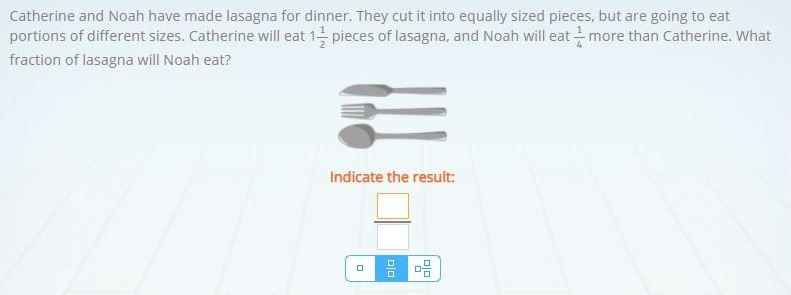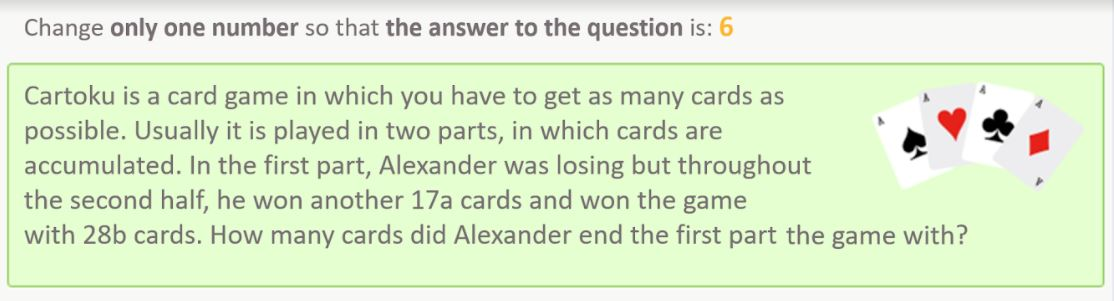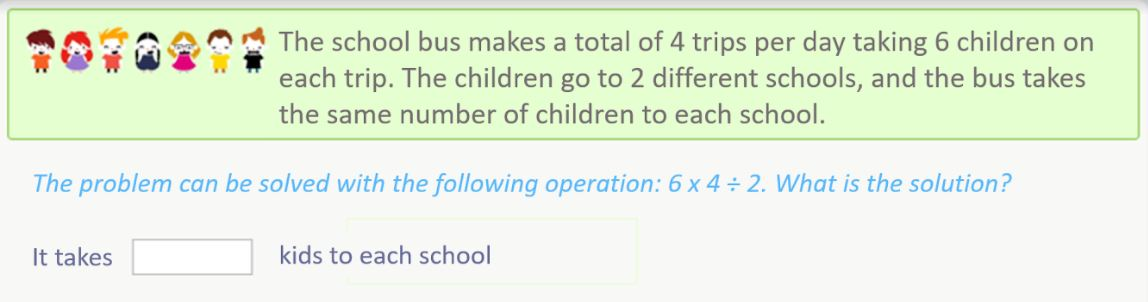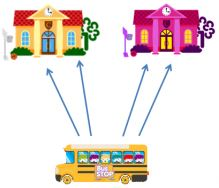Smartick is a fun way to learn math!Mar25

Examples of 3rd Grade Math Word Problems with Solutions

In this post, we are going to look at a selection of 3rd grade math word problems in Smartick.

We’ll begin by explaining what a word problem is.

Index

What is a Word Problem?

A word problem is a question or approach, that cannot be answered using one specific operation, but rather a combination of previous knowledge whether mathematical or not.

Solving a math problem is cognitively complex since it involves understanding the situation presented in the word problem, as well as comprehending the message and words used in the word problem. Therefore, at first, to face a math problem is to face a problem of linguistic understanding. Once the word problem is understood, you must choose which, or what, procedures you are going to use to reach a solution (mathematical understanding).

This type of activity helps a child to connect mathematics to the world around them and by doing this, they are able to apply what they have learned to everyday life. To solve a problem, it is not only necessary to master the specific knowledge related to the problem, but also plan and perform some procedures to reach the solution.

To assess mathematical competence in 3rd grade math word problems, there are some cognitive processes to keep in mind that fall into these three groups:

• Know and produce. It refers to the knowledge of the basic mathematical language, essential mathematical properties and facts, and the repetition of calculation algorithms practiced, linking them to familiar math processes and problems that include basic operations. This is required for even the most basic exercises.
• Apply and analyze. Involves knowing how to use different mathematical tools and establishing relationships between diverse situations in relatively well-known contexts, selecting problem-solving strategies, performing calculations, and obtaining solutions. This is necessary for exercises that are slightly more difficult.
• Reason and reflect. It requires the ability to think logically and systematically, and involves analyzing the results obtained in solving a problem and reflecting upon the process used. It refers to the interpretation of the results according to the context, communication of the method used, and the result obtained appropriately. It implies a higher degree of competitive acquisition.

Before starting practicing, I would recommend that you try to solve the 3rd grade math word problems on your own, without looking at the solutions. Give it a try!Solution 1

After reading the word problem we need to make sure that we understand the information given.

• The lasagna will be cut into equally sized pieces.
• Catherine and Noah will eat different sized portions.
• Noah will eat 1/4 more than Catherine.

Since we know that Catherine will eat a portion of 1 1/2 (1 full piece and one 1/2 piece) we just need to find out how large Noah’s portion will be. Let’s look at the fraction, how many times can 1/4 go into 1/2? Two times because there are 2 halves to a whole and 4 quarters to a whole, therefore 1/2 = 2/4. Now that we know Catherine will eat 1 2/4 and Noah will eat 1/4 more, 2/4 + 1/4 = 3/4.

Noah will eat 1 3/4 pieces of lasagna.

Word Problem 2Solution 2

We have been asked to change only one number in the word problem so that the number of cards that Alexander has at the end of the first part of the game is 6. We are told that he has won 17 cards during the second half and finishes the game with 28 cards. In order to know how many cards he had in the first part we should subtract what he has won during the second part from the total number of cards he ended the game with, in other words, 28 – 17 = 11. Since we have been asked to make sure the answer is 6 and can only change one data, we have two valid options.

• Change the number of cards he won during the second part, if he won 17 and we add 5, 17 +5 = 22. Then when we subtract that number from the total, 28 – 22 = 6, it gives us the number we need to solve the word problem. Alexander had 6 cards at the end of the first part of the game.
• Change the number of cards that he had at the end of the game, subtracting 5 from the 28 cards, 28 – 5 = 23. Then subtract the number of cards he won during the second part, 17, from the total, 23 – 17 = 6. Alexander had 6 cards at the end of the first part of the game.

Sometimes math problems have more than one solution.

Word Problem 3

Solution 3

This problem is very easy because the table shown has all of the information.

• The first thing we need to know is the total amount of sandwiches, of all varieties, served yesterday, both day and night. Put simply, we need to add 16 + 54 = 70 sandwiches.
• Next, we need to fill in the empty space for the Fisherman’s sandwich. In order to know how many were served in the evening, we need to subtract the number of sandwiches served during the day from the total.
• 54 – 27 = 27 Fisherman’s sandwiches we served yesterday evening in the cafeteria where Catherine works.

Word Problem 4Solution 4First of all, we want to find out the total number of children that are on the bus. To do this, we just need to complete a very simple operation. We know that there are 6 children on the bus and the bus makes 4 trips, so we can do 6 x 4 = 24. Now we know that there are a total of 24 children on the bus during the day. Next, we need to determine how many children are dropped off at each school. The word problem tells us that the same number of children are dropped off at each school, there are two schools, so let’s divide by 2!

24 / 2 = 12, the bus takes 12 children to each school.

If you would like to continue practicing more 3rd grade math word problems and other topics from primary mathematics, adapted to your level, register with Smartick and try it free!